# YVO4晶体的负折射特性分析与应用Analysis and Application of Negative Refraction of YVO4 Crystal

DOI: 10.12677/APP.2019.912062, PDF, HTML, XML, 下载: 293  浏览: 566

Abstract: This paper investigated the negative refraction details in YVO4 crystal in order to design new wave-guide. When e-light incidents on the interface of uniform media and uniaxial crystal, the refracted and incident rays may be on the same side of the normal line, which is negative refraction. The formula for e-light refraction was given in this paper. As a special uniaxial crystal with good optical characteristics and obvious birefrigence, YVO4 was researched in detail about the influence of the optical axis direction, the area of incident and refracted angles on negative refraction. And negative refraction can be used in stealth technology. In addition, twin-crystal structure was used to guide e-light to bypass some area or to bend some angle. The quantitative results of negative refraction and the twin crystal structure showed new lights on the application of negative refractions of YVO4 crystal.

1. 引言

YVO4晶体在可见光及近红外波段有良好的透光性，透光范围较宽，硬度较高，可人工生长出大块优质晶体，并且易于加工，价格较低，是光通信无源器件如光隔离器、旋光器、延迟器、偏振器中的关键材料。YVO4晶体(no = 2.02, ne = 2.25, 532 nm)相比KDP(no = 1.51, ne = 1.47, 532 nm)及其他光学晶体各向异性更强，负折射相关角度更大。为确定YVO4晶体的负折射与应用，本文详细分析YVO4晶体光轴取向不同时空气与晶体的界面上e光负折射的最大入射角与出射角范围及其与光轴角的关系，并分析e光在孪晶界面上的偏折特征。

2. YVO4晶体折射

3. e光在均匀介质与YVO4晶体界面的折射

$\mathrm{tan}{\theta }_{2}=\frac{1}{{n}_{o}^{2}{\mathrm{cos}}^{2}{\theta }_{0}+{n}_{e}^{2}{\mathrm{sin}}^{2}{\theta }_{0}}\left[\left({n}_{o}^{2}-{n}_{e}^{2}\right)\mathrm{sin}{\theta }_{0}\mathrm{cos}{\theta }_{0}+\frac{n{n}_{o}{n}_{e}\mathrm{sin}{\theta }_{1}}{\sqrt{{n}_{o}^{2}{\mathrm{cos}}^{2}{\theta }_{0}+{n}_{e}^{2}{\mathrm{sin}}^{2}{\theta }_{0}-{n}^{2}{\mathrm{sin}}^{2}{\theta }_{1}}}\right]$ (1)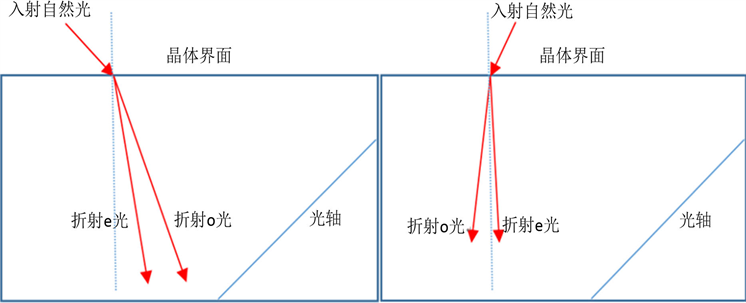Figure 1. Birefringence of natural light and negative refraction of e-ray on interface of uniaxial crystal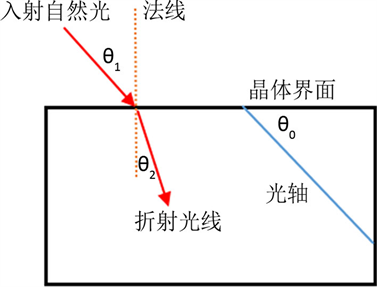Figure 2. Angles on interface of uniform and uniaxial media

${\theta }_{1m}=\mathrm{arcsin}\left[\sqrt{\frac{\left({n}_{e}^{2}-{n}_{o}^{2}\right){\mathrm{sin}}^{2}{\theta }_{0}{\mathrm{cos}}^{2}{\theta }_{0}}{{n}^{2}\left({n}_{e}^{2}{\mathrm{cos}}^{2}{\theta }_{0}+{n}_{o}^{2}{\mathrm{sin}}^{2}{\theta }_{0}\right)}}\right]$ (2)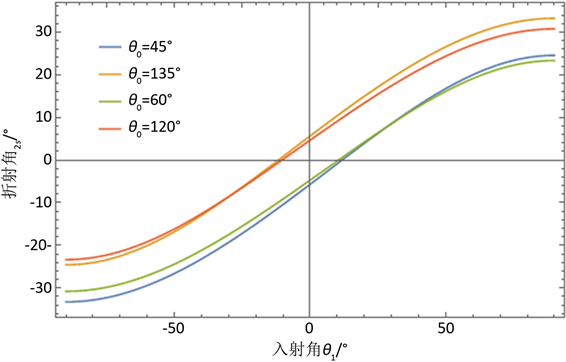Figure 3. Refractive angles of e-ray on air-YVO4 interface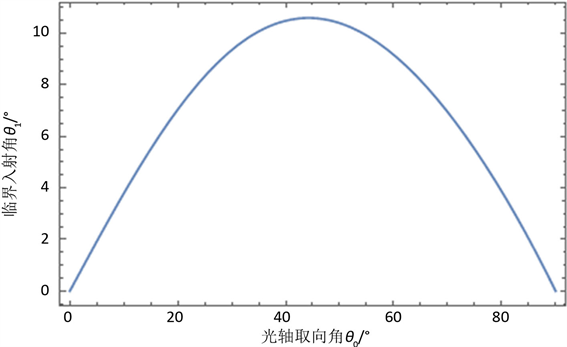Figure 4. Relation of critical incident angle and the axial angle

${\theta }_{1m\mathrm{max}}=\mathrm{arcsin}\left(\frac{{n}_{e}-{n}_{o}}{n}\right)$ (3)

YVO4晶体的最佳光轴取向角约为46.43˚，最大临界角为11.5˚。负折射的临界入射角最大值取决于晶体双折射率 $|{n}_{e}-{n}_{o}|/n$，晶体双折射率越大临界入射角最大值越大。

${\theta }_{2sm}=\mathrm{arctan}\left[\frac{\left({n}_{o}^{2}-{n}_{e}^{2}\right)\mathrm{sin}{\theta }_{0}\mathrm{cos}{\theta }_{0}}{{n}_{o}^{2}{\mathrm{cos}}^{2}{\theta }_{0}+{n}_{e}^{2}{\mathrm{sin}}^{2}{\theta }_{0}}\right]$ (4)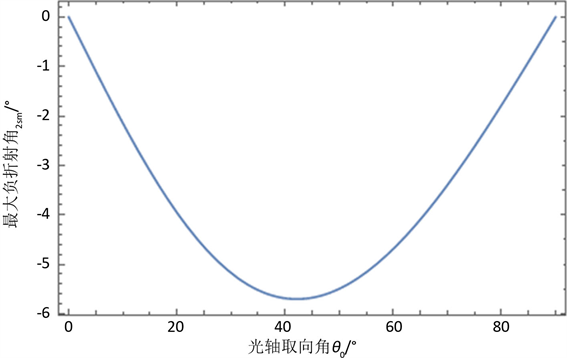Figure 5. Relation of maximum negative refractive angle and axial angle

${\theta }_{0}=\text{arcsin}\left[\sqrt{{n}_{o}^{2}/\left({n}_{o}^{2}+{n}_{e}^{2}\right)}\right]$ 时最大负折射角为：

${\theta }_{2s\mathrm{max}}=\mathrm{arctan}\left[\frac{{n}_{e}^{2}-{n}_{o}^{2}}{2{n}_{o}{n}_{e}}\right]$ (5)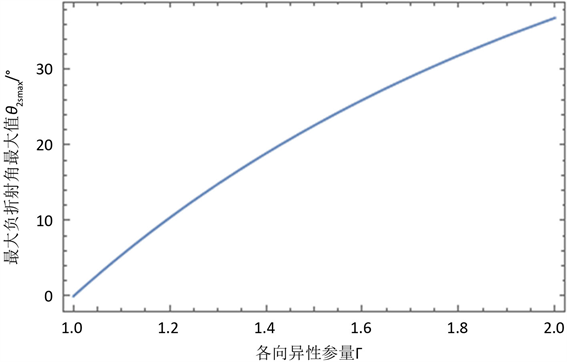Figure 6. Relation of maximum negative refractive angle and anisotropic parameter

4. 结论与应用分析

YVO4晶体e光负折射实现一定角度范围隐身，折射光线偏离正折射情况导致一定范围内的目标在视场内消失。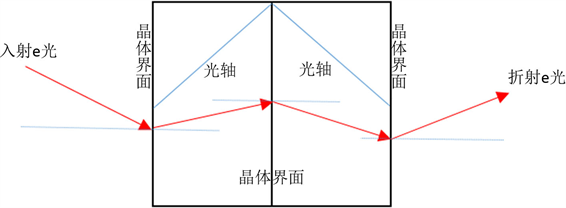Figure 7. Route of light across the twin-crystal structure

  Talalai, G.A., Garner, T.J. and Weiss, S.J. (2018) Explaining Negative Refraction without Negative Refractive Indices. Journal of the Optical Society of America A, 35, 437-441. https://doi.org/10.1364/JOSAA.35.000437  Chen, X.L., He, M. and Du, Y.X. (2005) Negative Refraction: An Intrinsic Property of Uniaxial Crystals. Physical Review B, 72, Article ID: 113111. https://doi.org/10.1103/PhysRevB.72.113111  Ren, Q., Zhan, Q.W. and Liu, Q.H. (2017) An Improved Subdomain Level Nonconformal Discontinuous Galerkin Time Domain (DGTD) Method for Materials with Full-Tensor Constitutive Parameters. IEEE Photonics Journal, 9, 1-13. https://doi.org/10.1109/JPHOT.2017.2672644  Veselago, V. (1968) The Electrodynamics of Substances with Simultaneously Negative Values of ε and μ. Soviet Physics Uspekhi, 10, 509-514. https://doi.org/10.1070/PU1968v010n04ABEH003699  Shelby, R.A., Smith, D.R. and Schultz, S. (2001) Experimental Verification of a Negative Index of Refraction. Science, 292, 77-79. https://doi.org/10.1126/science.1058847  Zhang, Y. and Mascarenhas, A. (2007) Negative Refraction of Electromagnetic and Electronic Waves in Uniform Media. In: Krowne, C.M. and Zhang, Y., Eds., Physics of Negative Refraction and Negative Index Materials: Optical and Electronic Aspects and Diversified Approaches, Springer, Berlin, 8-12. https://doi.org/10.1007/978-3-540-72132-1_1  何金龙. 负折射介质波导特性及应用研究[D]: [博士学位论文]. 杭州: 浙江大学, 2006.  杜银霄, 张又林, 麻华丽, 等. 各向异性晶体的平板负折射成像研究[J]. 光学学报, 2013, 33(6): 1-7.  Wang, W.H., Cui, X.D., Yang, E.C., et al. (2015) Negative Refraction in Molybdenum Disulfide. Optics Express, 23, 22024-22033. https://doi.org/10.1364/OE.23.022024  Zhang, Y., Fluegel, B. and Mascarenhas, A. (2003) Total Negative Refraction in Real Crystals for Ballistic Electrons and Light. Physical Review Letters, 95, Article ID: 157404. https://doi.org/10.1103/PhysRevLett.91.157404  鲁拥华, 王沛, 姚培军, 等. 利用单轴晶体实现的负折射现象[J]. 液晶与显示, 2004, 19(4): 304-307.  宋哲, 郝林岗, 吴宁, 等. 光轴取向任意时单轴晶体内表面上的双反射[J]. 辽宁师范大学学报(自然科学版), 2013, 36(2): 168-172.  宋哲, 郝林岗, 吴宁, 等. 光轴取向任意时单轴晶体界面上的双折射[J]. 辽宁师范大学学报(自然科学版), 2013, 36(3): 335-339.  刘才明. 单轴晶体内非寻常光折射方向的普遍规律[J]. 光电工程, 2003, 30(2): 25-42.  罗海陆, 胡巍, 罗煦农, 等. 单轴晶体中的负折射现象研究[J]. 光学学报, 2005, 25(9): 1250-1252.